# Jakub

Jakub multiplied 11 times the number 100 by ten and then divided the result ten times by 10. What number did he get?

n =  1000

### Step-by-step explanation: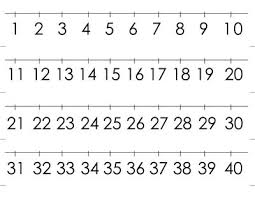Did you find an error or inaccuracy? Feel free to write us. Thank you!Tips to related online calculators
Do you want to perform natural numbers division - find the quotient and remainder?

## Related math problems and questions:

• RemaindersIt is given a set of numbers { 170; 244; 299; 333; 351; 391; 423; 644 }. Divide this numbers by number 66 and determine set of remainders. As result write sum of this remainders.
• The resultHow many times I decrease the number 1632 to get the result 24?
• Animal species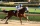Of 100 types of animals, 9/100 were discovered in ancient times, and 2/100 were discovered in the Middle Ages. Another 3/10 were discovered in the 1800s. What fraction of the 100 types of animals was discovered after the 1800s? Explain.
• Quotient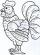Determine the quotient (q) and the remainder (r) from division numbers 100 and 8. Take the test of correctness.
• Two hundred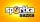Two hundred ten athletes competed in three athletics races on three fields. One hundred five athletes competed in the first, 60 in the second, and everyone else in the third. On the individual courts, the athletes were divided into groups. Each group, alt
• I thinkI think a number. When I multiply it by five, and after that I subtract 477, I get the same number as if I multiplied it twice. What number do I think?
• Big number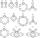The hat is the remainder when dividing number 10 to 47 - 111 by number 9?
• DivisibilityIs the number 761082 exactly divisible by 9? (the result is the integer and/or remainder is zero)
• Buildings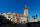The school stands 110 m from the church, which is 100 m beyond the town hall. Which building are closer to the church and how many times?
• MistakeNicol mistake when calculate in school. Instead of add number 20 subtract it. What is the difference between the result and the right result?
• Unknown numberIf I reduced the sum of the numbers 70 and the unknown number three times, I would get 100. what is the unknown number?
• Unknown number 16My number's tens is three times more then ones My number's ones is twice the number of thousands and my number's hundreds is half the number of of tens. I have two ones. which number am I?
• Unknown numberSamuel wrote unknown number. Then he had add 200000 to the number and the result multiply by three. When it calculated he was surprised, because the result would have received anyway, if write digit to the end of original number. Find unknown number.
• NumberCalculate the integer number which, divided by 34 gives 10 and the rest 25.
• Number unknownAdela thought the two-digit number, she added it to its ten times and got 407. What number does she think?
• DivisionWhich number in division 16 give 12 and the rest 3?
• Chocolate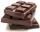How many times do we have to break a chocolate bar composed of 10 × 12 pieces to get the 120 parts?